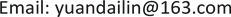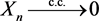﻿ 随机变量序列完全收敛的等价及充分条件 Equivalent and Sufficient Conditions of Complete Convergence of Random Variables Sequence

Vol.07 No.06(2018), Article ID:25477,7 pages
10.12677/AAM.2018.76080

Equivalent and Sufficient Conditions of Complete Convergence of Random Variables Sequence

Dailin Yuan

School of Mathematics, Southwest Jiaotong University, Chengdu SichuanReceived: May 24th, 2018; accepted: Jun. 12th, 2018; published: Jun. 19th, 2018ABSTRACT

The equivalent condition and two sufficient conditions of complete convergence were obtained based on a monotone decreasing real sequence converging to 0, when the convergence of a random variables sequence was considered. At the same time, the related issues about almost sure convergence and convergence in probability were researched. The interrelations of these conditions were obtained and exemplified.

Keywords:Complete Convergence, Equivalent Condition, Sufficient Condition1. 引言

2. 随机变量序列收敛定义及相关定理

$\underset{n\to \infty }{\mathrm{lim}}P\left(|{X}_{n}-X|>\epsilon \right)=0,\text{\hspace{0.17em}}\text{\hspace{0.17em}}\forall \epsilon >0$ (1)

$P\left(\underset{n\to \infty }{\mathrm{lim}}{X}_{n}=X\right)=1$ (2)

$\sum _{n=1}^{\infty }\text{ }P\left(|{X}_{n}-X|>\epsilon \right)<\infty ,\text{\hspace{0.17em}}\text{\hspace{0.17em}}\forall \epsilon >0$ (3)

${X}_{n}\stackrel{\text{c}\text{.c}\text{.}}{\to }X⇒{X}_{n}\stackrel{\text{a}\text{.s}\text{.}}{\to }X⇒{X}_{n}\stackrel{p}{\to }X$

$P\left(\underset{n=1}{\overset{\infty }{\cap }}\underset{m=n}{\overset{\infty }{\cup }}\left\{|{X}_{m}-X|>\epsilon \right\}\right)=0,\text{\hspace{0.17em}}\text{\hspace{0.17em}}\forall \epsilon >0$ (4)

$\underset{n\to \infty }{\mathrm{lim}}P\left(\underset{m=n}{\overset{\infty }{\cup }}\left\{|{X}_{m}-X|>\epsilon \right\}\right)=0,\text{\hspace{0.17em}}\text{\hspace{0.17em}}\forall \epsilon >0$ (5)

$P\left(\underset{k=1}{\overset{\infty }{\cup }}\underset{n=1}{\overset{\infty }{\cap }}\underset{m=n}{\overset{\infty }{\cup }}\left\{|{X}_{m}-X|>\frac{1}{k}\right\}\right)=0$ (6)

$\underset{k\to \infty }{\mathrm{lim}}P\left(\underset{n=1}{\overset{\infty }{\cap }}\underset{m=n}{\overset{\infty }{\cup }}\left\{|{X}_{m}-X|>\frac{1}{k}\right\}\right)=0$ (7)

$\underset{k\to \infty }{\mathrm{lim}}\underset{n\to \infty }{\mathrm{lim}}P\left(\underset{m=n}{\overset{\infty }{\cup }}\left\{|{X}_{m}-X|>\frac{1}{k}\right\}\right)=0$ (8)

$\underset{k=1}{\overset{\infty }{\cup }}\underset{n=1}{\overset{\infty }{\cap }}\underset{m=n}{\overset{\infty }{\cup }}\left\{|{X}_{m}-X|>\frac{1}{k}\right\}=\underset{k=1}{\overset{\infty }{\cup }}\underset{n=1}{\overset{\infty }{\cap }}\underset{m=n}{\overset{\infty }{\cup }}\left\{|{X}_{m}-X|>{\epsilon }_{k}\right\}$

$\exists {\epsilon }_{k}↓0,\text{\hspace{0.17em}}\text{s}\text{.t}\text{.}\text{\hspace{0.17em}}P\left(\underset{k=1}{\overset{\infty }{\cup }}\underset{n=1}{\overset{\infty }{\cap }}\underset{m=n}{\overset{\infty }{\cup }}\left\{|{X}_{m}-X|>{\epsilon }_{k}\right\}\right)=0$ (9)

$\exists {\epsilon }_{k}↓0,\text{\hspace{0.17em}}\text{s}\text{.t}\text{.}\text{\hspace{0.17em}}\underset{k\to \infty }{\mathrm{lim}}P\left(\underset{n=1}{\overset{\infty }{\cap }}\underset{m=n}{\overset{\infty }{\cup }}\left\{|{X}_{m}-X|>{\epsilon }_{k}\right\}\right)=0$ (10)

$\exists {\epsilon }_{k}↓0,\text{\hspace{0.17em}}\text{s}\text{.t}\text{.}\text{\hspace{0.17em}}\underset{k\to \infty }{\mathrm{lim}}\underset{n\to \infty }{\mathrm{lim}}P\left(\underset{m=n}{\overset{\infty }{\cup }}\left\{|{X}_{m}-X|>{\epsilon }_{k}\right\}\right)=0$ (11)

$\exists {\epsilon }_{k}↓0,\text{\hspace{0.17em}}\text{s}\text{.t}\text{.}\text{\hspace{0.17em}}\underset{n\to \infty }{\mathrm{lim}}P\left(\underset{k=n}{\overset{\infty }{\cup }}\left\{|{X}_{k}-X|>{\epsilon }_{k}\right\}\right)=0$ (12)

$\exists {\epsilon }_{k}↓0,\text{\hspace{0.17em}}\text{s}\text{.t}\text{.}\text{\hspace{0.17em}}P\left(\underset{n=1}{\overset{\infty }{\cap }}\underset{k=n}{\overset{\infty }{\cup }}\left\{|{X}_{k}-X|>{\epsilon }_{k}\right\}\right)=0$ (13)

3. 主要结果及证明

$\exists {\epsilon }_{n}↓0,\text{\hspace{0.17em}}\text{s}\text{.t}\text{.}\text{\hspace{0.17em}}\sum _{n=1}^{\infty }P\left(|{X}_{n}-X|>{\epsilon }_{n}\right)<\infty$ (14)

$\begin{array}{c}\sum _{n=1}^{\infty }P\left(|{X}_{n}-X|>\epsilon \right)\le \sum _{n=1}^{N}P\left(|{X}_{n}-X|>\epsilon \right)+\sum _{n=N+1}^{\infty }P\left(|{X}_{n}-X|>{\epsilon }_{n}\right)\\ \le \sum _{n=1}^{N}P\left(|{X}_{n}-X|>\epsilon \right)+\sum _{n=1}^{\infty }P\left(|{X}_{n}-X|>{\epsilon }_{n}\right)<\infty \end{array}$

${X}_{n}\stackrel{\text{c}\text{.c}\text{.}}{\to }X$

“⟹”：若 ${X}_{n}\stackrel{\text{c}\text{.c}\text{.}}{\to }X$ ，取实数序列 $\left\{{\eta }_{n}\right\}$${\eta }_{n}↓0$ ，则对 ${\eta }_{1}>0$ ，有

${M}_{1}=\sum _{n=1}^{\infty }P\left(|{X}_{n}-X|>{\eta }_{1}\right)<\infty$

$\sum _{n=1}^{\infty }P\left(|{X}_{n}-X|>{\eta }_{2}\right)<\infty$

$\sum _{n={N}_{1}+1}^{\infty }P\left(|{X}_{n}-X|>{\eta }_{2}\right)<\frac{1}{{2}^{2}}$

$\sum _{n=1}^{{N}_{1}}P\left(|{X}_{n}-X|>{\eta }_{1}\right)+\sum _{n={N}_{1}+1}^{\infty }P\left(|{X}_{n}-X|>{\eta }_{2}\right)<{M}_{1}+\frac{1}{{2}^{2}}$

$\sum _{n={N}_{k-1}+1}^{\infty }P\left(|{X}_{n}-X|>{\eta }_{k}\right)<\frac{1}{{k}^{2}}$

${\eta }_{k+1}>0$ ，存在正整数 ${N}_{k}$ ，且满足 ${N}_{k-1}+1\le {N}_{k}$ ，使得

$\sum _{n={N}_{k}+1}^{\infty }P\left(|{X}_{n}-X|>{\eta }_{k+1}\right)<\frac{1}{{\left(k+1\right)}^{2}}$

$\sum _{n={N}_{k-1}+1}^{{N}_{k}}P\left(|{X}_{n}-X|>{\eta }_{k}\right)\le \sum _{n={N}_{k-1}+1}^{\infty }P\left(|{X}_{n}-X|>{\eta }_{k}\right)<\frac{1}{{k}^{2}}$

${N}_{0}=0$ ，有

$\sum _{k=1}^{\infty }\sum _{n={N}_{k-1}+1}^{{N}_{k}}P\left(|{X}_{n}-X|>{\eta }_{k}\right)<{M}_{1}+\sum _{k=1}^{\infty }\frac{1}{{k}^{2}}<\infty$

$\sum _{n=1}^{\infty }P\left(|{X}_{n}-X|>{\epsilon }_{n}\right)=\sum _{k=1}^{\infty }\sum _{n={N}_{k-1}+1}^{{N}_{k}}P\left(|{X}_{n}-X|>{\eta }_{k}\right)<{M}_{1}+\sum _{k=1}^{\infty }\frac{1}{{k}^{2}}<\infty$ 。 □

$f\left(x\right)=\left\{\begin{array}{l}{|x|}^{-3},\text{\hspace{0.17em}}\text{\hspace{0.17em}}当|x|>1\\ 0,\text{\hspace{0.17em}}\text{\hspace{0.17em}}\text{\hspace{0.17em}}\text{\hspace{0.17em}}\text{\hspace{0.17em}}\text{\hspace{0.17em}}\text{\hspace{0.17em}}其它\end{array}$

$E|\xi |=2<\infty$$E\left(\xi \right)=0$$E\left({\xi }^{2}\right)=\infty$ 。令 ${X}_{n}=\frac{{\xi }_{1}+{\xi }_{2}\cdots +{\xi }_{n}}{n}$ ，由Kolmogorov强大数定律(  , p. 295)， ${X}_{n}\stackrel{\text{a}\text{.s}\text{.}}{\to }0⇔E|\xi |<\infty$$E\left(\xi \right)=0$ ；由Hsu-Robbins-Erdos强大数定律(  ，p312)， ${X}_{n}\stackrel{\text{c}\text{.c}\text{.}}{\to }0⇔E\left({\xi }^{2}\right)<\infty$$E\left(\xi \right)=0$ 。由此知 ${X}_{n}\stackrel{\text{a}\text{.s}\text{.}}{\to }0$ ，但不成立。

$\sum _{n=1}^{\infty }P\left(|{X}_{n}-0|>\epsilon \right)=\sum _{n=1}^{\infty }P\left({X}_{n}=1\right)=\sum _{n=1}^{\infty }\frac{1}{{n}^{2}}=\infty$

$\exists {\epsilon }_{k}↓0,\text{\hspace{0.17em}}\text{s}\text{.t}\text{.}\text{\hspace{0.17em}}P\left(|{X}_{n}-X|>{\epsilon }_{k}\right)=0,\text{\hspace{0.17em}}\text{\hspace{0.17em}}\text{for}\text{\hspace{0.17em}}\text{all}\text{\hspace{0.17em}}{\epsilon }_{k}$ (15)。简记为 ${X}_{n}\stackrel{\text{a}\text{.s}.}{=}X,\text{\hspace{0.17em}}\forall n$

$\begin{array}{c}P\left({X}_{n}\ne X\right)=P\left(|{X}_{n}-X|\ne 0\right)\\ =P\left(\underset{k=1}{\overset{\infty }{\cup }}\left\{|{X}_{n}-X|>{\epsilon }_{k}\right\}\right)\\ \le \sum _{k=1}^{\infty }P\left(|{X}_{n}-X|>{\epsilon }_{k}\right)=0\end{array}$

$P\left({X}_{n}=X\right)=1-P\left({X}_{n}\ne X\right)=1-0=1$ . □

$\exists {\epsilon }_{n}↓0,\text{\hspace{0.17em}}\text{s}\text{.t}\text{.}\text{\hspace{0.17em}}P\left(|{X}_{n}-X|>{\epsilon }_{n}\right)=0$ (16)

$\begin{array}{c}\sum _{n=1}^{\infty }P\left(|{X}_{n}-X|>\epsilon \right)\le \sum _{n=1}^{N}P\left(|{X}_{n}-X|>\epsilon \right)+\sum _{n=N+1}^{\infty }P\left(|{X}_{n}-X|>{\epsilon }_{n}\right)\\ =\sum _{n=1}^{N}P\left(|{X}_{n}-X|>\epsilon \right)+0<\infty \end{array}$

$P\left(\underset{m=n}{\overset{\infty }{\cup }}\left\{|{X}_{m}-X|>\epsilon \right\}\right)\le \sum _{m=n}^{\infty }P\left(|{X}_{m}-X|>\epsilon \right)\le \sum _{m=n}^{\infty }P\left(|{X}_{m}-X|>{\epsilon }_{m}\right)=0$

$\underset{n\to \infty }{\mathrm{lim}}P\left(\underset{m=n}{\overset{\infty }{\cup }}\left\{|{X}_{m}-X|>\epsilon \right\}\right)=0$

$P\left({X}_{n}=0\right)=1-\frac{1}{{n}^{2}},\text{\hspace{0.17em}}\text{\hspace{0.17em}}P\left({X}_{n}=1\right)=\frac{1}{{n}^{2}}$

$\sum _{n=1}^{\infty }P\left(|{X}_{n}-0|>\epsilon \right)=\sum _{n=1}^{\infty }P\left({X}_{n}=1\right)=\sum _{n=1}^{\infty }\frac{1}{{n}^{2}}<\infty$

${X}_{n}\stackrel{\text{a}\text{.s}.}{=}X$ ⟺(15)⇒(16)⇒(14)⟺ ${X}_{n}\stackrel{\text{c}\text{.c}}{\to }X$${X}_{n}\stackrel{\text{a}\text{.s}\text{.}}{\to }X$ ⟺(12)⇒ ${X}_{n}\stackrel{p}{\to }X$

Equivalent and Sufficient Conditions of Complete Convergence of Random Variables Sequence[J]. 应用数学进展, 2018, 07(06): 673-679. https://doi.org/10.12677/AAM.2018.76080

1. 1. Gut, A. (2005) Probability: A Graduate Course. Springer, New York.

2. 2. 蔡光辉. 混合序列的矩不等式与完全收敛性[J]. 系统科学与数学, 2008, 28(2): 251-256.

3. 3. Huang, H.W. (2013) Complete Convergence of Pairwise NQD Random Sequences. Chinese Journal of Applied Probability and Statistics, 29, 275-286.

4. 4. Shen, Y., Wang, X.J., Hu, S.H. and Yang, W.Z. (2010) Convergence Properties of -Mixing Random Variable Sequences. Journal of University of Science and Technology of China, 40, 914-919.

5. 5. Wu, Q.Y. (2008) Almost Sure Convergence for φ-mixing Random Variable Sequences. Mathematica Applicata, 21, 629-634.

6. 6. Huang, H.W., Wang, D.C. and Wu, Q.Y. (2012) Strong Convergence Laws for -Mixing Sequences of Random Variables. Chinese Journal of Applied Probability and Statistics, 28, 181-188.

7. 7. Loeve, M. (1977) Probability Theory I. Springer, New York.